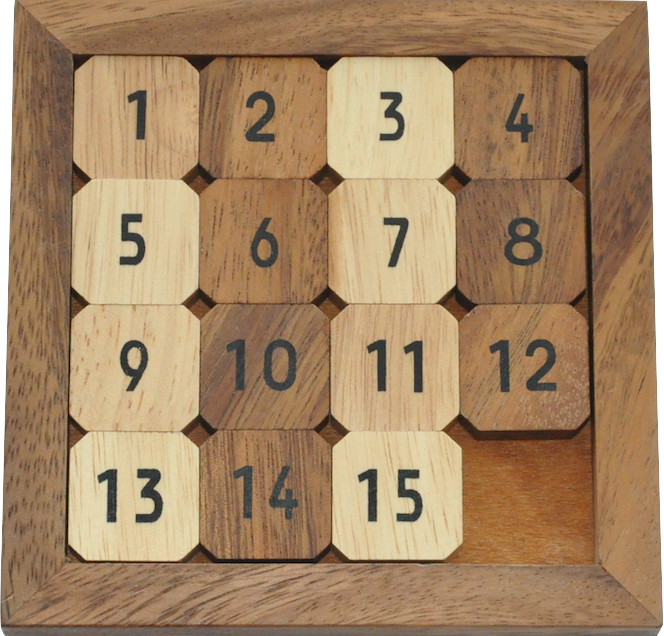# 15-puzzle ($n^2-1$ puzzle)

$n^2-1$ numbered tiles can be slid in a $n \times n$ board with the goal of arranging them in increasing order.## Description

$n^2-1$ of the $n^2$ positions a $n \times n$ matrix are filled by tiles, leaving one unfilled hole. A move consists of sliding a tile that is adjacent to the hole into the hole (effectively swapping them). The goal is to rearrange the tiles into a particular permutation.

Typically tiles are numbered with the integers from $1$ to $n^2-1$ and the goal is to arrange them in increasing order (left to right, top to bottom) with the hole being on the bottom right corner.

## Computational complexity

Determining whether a solution exists is in P  .

Finding the shortest solution is NP-hard   and in APX .

 A. F. Archer, “A Modern Treatment of the 15 Puzzle”, The American Mathematical Monthly, 2000.

 D. Kornhauser, G. Miller, P. Spirakis, “Coordinating Pebble Motion On Graphs, The Diameter Of Permutation Groups, And Applications”, in FOCS 1984.

 O. Goldreich, “Finding the Shortest Move-Sequence in the Graph-Generalized 15-Puzzle Is NP-Hard”, Studies in Complexity and Cryptography, 2011.

 E. D. Demaine, M. Rudoy, “A simple proof that the ($n^2$ − 1)-puzzle is hard”, Theoretical Computer Science, 2018.

 D. Ratner, M. Warrnuth, “Finding a Shortest Solution for the n x n extension of the 15-Puzzle is Intractable”, in AAAI 1986.

@misc{cog:15-puzzle,
author = "{CoG contributors}",
title  = "{15-puzzle ($n^2-1$ puzzle) --- Complexity of Games}",
year   = "2022",
url    = "https://www.isnphard.com/i/15-puzzle/",
note   = "[Online; accessed 2022-06-02]"
}